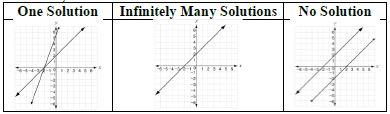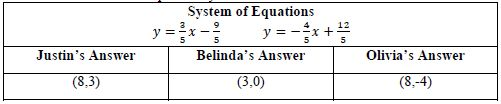# Standard #: MA.8.AR.4.1

This document was generated on CPALMS - www.cpalms.org

Given a system of two linear equations and a specified set of possible solutions, determine which ordered pairs satisfy the system of linear equations.

### Clarifications

Clarification 1: Instruction focuses on the understanding that a solution to a system of equations satisfies both linear equations simultaneously.

### General Information

Subject Area: Mathematics (B.E.S.T.)
Strand: Algebraic Reasoning
Status: State Board Approved

### Terms from the K-12 Glossary

• Linear Equation

### Vertical Alignment

Previous Benchmarks

Next Benchmarks

### Purpose and Instructional Strategies

In grade 7, students determined constants of proportionality and graphed proportional relationships from a table, equation or a written description given in a mathematical or real-world context. In grade 8, students extend this learning to systems of two linear equations to determine possible solutions from a specified set of ordered pairs. In Algebra 1, students will write and solve a system of two-variable linear equations algebraically and graphically given a mathematical or real-world context.
• The focus of this benchmark is on the understanding that the solution of a system is a set of points that satisfy both equations of the system.
• Systems of linear equations can have one solution, infinitely many solutions or no solutions.
• A system of linear equations whose graphs meet at one point (intersecting lines) has only one solution, the ordered pair representing the point of intersection.
• A system of linear equations whose graphs are coincident (the same line) has infinitely many solutions, the set of ordered pairs representing all the points on the line.
• A system of linear equations whose graphs do not meet (parallel lines) has no solutions and the slopes of these lines are the same. The technical name for these kinds of systems is "inconsistent".• A system of linear equations is two linear equations that should be solved at the same time.
• Instruction includes understanding that systems are on the same coordinate plane to determine solutions (MTR.4.1).

### Common Misconceptions or Errors

• Students may incorrectly substitute solutions into the equations. To address this misconception, remediate work with integers.
• Students may not understand how a pair of values can be a single solution to a pair of equations. Emphasize the connection between the two variables in the equations and the two coordinates of the point on the coordinate plane.

### Strategies to Support Tiered Instruction

• Instruction includes drawing connections between systems of equations represented graphically and with equations. Using a graphic organizer, reinforce the solution to a system of equation as the ordered pair that satisfies both equations simultaneously.
• When there is one solution, the two lines intersect at one point and when using substitution, the coordinates of that one point will result in true statements for both equations.
• When there is no solution, the two lines do not intersect and therefore there are no coordinates that will result in true statements for both equations.
• When there are infinite solutions, the two lines coincide and intersect with an infinite number of points. When using substitution, all the points on the lines will results in true statements for both equations.
• Teacher provides opportunities to utilize manipulatives when substituting values into given equations in order to help visualize evaluating operations with integers.
• 4 + 3, both positive, sum is positive. When the signs are the same the counters would be the same color, so add them.
• −4 + 3, different signs, more negative counters, the sum is negative.
• −4 + (−3), both negative signs, the sum is negative.
• 4 + (−3), different signs, more positive counters, the sum is positive.
• Teacher provides scaffolding opportunities by first having students identify which value in each ordered pair represents the $x$-coordinate and which represents the $y$-coordinate. Once students correctly identify the coordinates, the teacher provides opportunities to use substitution to replace the variables in the provided equations with the correct value. Finally, students evaluate each equation to determine if the provided coordinates result in true statements or not.
• Instruction includes remediating work with integers for students that incorrectly substitute solutions into the equations.

Instructional Task 1 (MTR.7.1, MTR 6.1)
The students in Mr. Cruz's Algebra class were determining the solution of a system of equations and determined what they believe are possible solutions. When Mr. Cruz checked their solutions, each of the students had a different set of points. Determine which student has the correct solution and explain why the other student's answers are not correct.### Instructional Items

Instructional Item 1
Determine which of the following points are a solution(s) of the given system of equations.
2$x$ − 8 = $y$ $x$ + 1 = $y$
a. (2, 2)
b. (3,2)
c. (0, 2)
d. (3, −2)
e. (3,3)

*The strategies, tasks and items included in the B1G-M are examples and should not be considered comprehensive.

#### Related Courses

 Course Number1111 Course Title222 1205050: M/J Accelerated Mathematics Grade 7 (Specifically in versions: 2014 - 2015, 2015 - 2020, 2020 - 2022, 2022 and beyond (current)) 1205070: M/J Grade 8 Pre-Algebra (Specifically in versions: 2014 - 2015, 2015 - 2022, 2022 and beyond (current)) 1204000: M/J Foundational Skills in Mathematics 6-8 (Specifically in versions: 2014 - 2015, 2015 - 2022, 2022 and beyond (current)) 7812030: Access M/J Grade 8 Pre-Algebra (Specifically in versions: 2014 - 2015, 2015 - 2018, 2018 - 2019, 2019 - 2022, 2022 and beyond (current))

#### Related Access Points

 Access Point Number Access Point Title MA.8.AR.4.AP.1a Given a system of two linear equations displayed on a graph, identify the solution of a system as the point where the two lines intersect. MA.8.AR.4.AP.1b Identify the coordinates of the point of intersection for two linear equations plotted on a coordinate plane.

Printed On:10/3/2023 10:10:49 AM
Print Page | Close this window